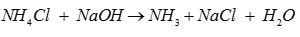# The p-Block Elements - Online Test

Q1. Elements having +5 oxidation state is
Explaination / Solution:

The maximum oxidation state shown by a p-block element is equal to the total number of valence electrons (i.e., the sum of the s and p-electrons).

P:[Ne]3s23p3. Total valence electrons =5 so it will show +5 oxidation state. The stability of +5 oxidation state decreases down the group due to inert pair effect.

Q2. [Ar] 3d104s24p3 is the electronic configuration of
Explaination / Solution:

Arsenic is a group 15 element and its atomic no. is 33.

Q3. The most common oxidation states of nitrogen are
Explaination / Solution:

N shows -3 in NH3 , +3 in HNO2 and +5 in HNO3.
Q4. In which of the following compound, nitrogen shows the oxidation state of +5?
Explaination / Solution:

Oxidation state of O is -2 and H is +1. Let the oxidation state of N is x.

(+1) +x + 3(-2) = 0

x = +5

Q5. The maximum covalency of nitrogen is
Explaination / Solution:

N has maximum covalency of 4 since only four (one s and three p) orbitals are available for bonding. d orbitals are not available for bonding.

Q6. Which among the following forms basic oxide?
Explaination / Solution:

As we move down the group in periodic table , metallic character increases so Bi is a metal thus its oxide is basic.

Q7. In laboratory ammonia is prepared by heating
Explaination / Solution:Q8. Ammonia has a higher boiling point and is less volatile because of
Explaination / Solution:

In the solid and liquid states NH3 is associated through  intermolecular hydrogen bonding and therefore it has higher boiling point and less volatile compared to other hydrides of group 15.
Q9. Which compound is used in the preparation of caprolactam?
Explaination / Solution:

NH3 is used in preparation of caprolactum. Caprolactam is an organic compound with the formula (CH2)5C(O)NH. It is used to make Nylon 6 fiber and plastics.
Q10. Which compound is used as the cooling liquid in refrigerators?
Explaination / Solution:

NH3 is used as cooling liquid in refrigerators